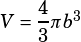I’m having problems with question #2 P.284
and I’ve checked the solution on page 349 but I still don’t get itOK, so in the solution in the book I approach this by reconciling the given equation with the general equation for the volume of a sphere (). If that’s not resonating with you, maybe think about it this way.

Start with a sphere of radius b. That has a volume of. Now imagine another, larger sphere with radius a being placed around that sphere, sharing the same center. Hard for me to draw spheres but here’s a 2-d circle version:

OK so far?

Now imagine that these aren’t full spheres, but half spheres. And then imagine that the sphere with radius b isn’t solid; it’s just empty space. So you’re left with just the shell (the bowl, in this problem).

The maximum amount of liquid the bowl can hold is the volume of the smaller sphere with radius b. That’s going to be half of the volume we figured out above for the sphere with radius b.Does that help?